E denotes electric field in a uniform conductor, I corresponding current through it, Vd drifts velocity of electrons and P denotes thermal power produced in the conductor, then which of the following graph is incorrect :

(1)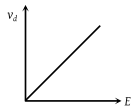(2)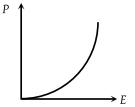(3)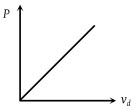(4)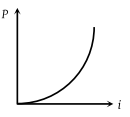Concept Questions :-

Current and current density
High Yielding Test Series + Question Bank - NEET 2020

Difficulty Level:

The two ends of a uniform conductor are joined to a cell of e.m.f. E and some internal resistance. Starting from the midpoint P of the conductor, we move in the direction of current and return to P. The potential V at every point on the path is plotted against the distance covered (x). Which of the following graphs best represents the resulting curve

(1)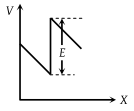(2)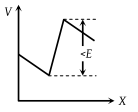(3)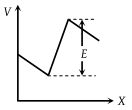(4)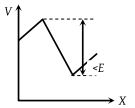Concept Questions :-

Emf and terminal voltage
High Yielding Test Series + Question Bank - NEET 2020

Difficulty Level:

The resistance Rt of a conductor varies with temperature t as shown in the figure. If the variation is represented by ${R}_{t}={R}_{0}\left[1+\alpha t+\beta {t}^{2}\right]$, then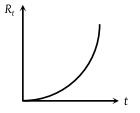(1) α and β are both negative

(2) α and β are both positive

(3) α is positive and β is negative

(4) α is negative and β are positive

Concept Questions :-

Derivation of Ohm's law
High Yielding Test Series + Question Bank - NEET 2020

Difficulty Level:

Variation of current and voltage in a conductor has been shown in the diagram below. The resistance of the conductor is :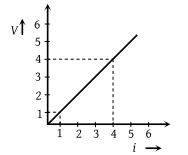(1) 4 ohm

(2) 2 ohm

(3) 3 ohm

(4) 1 ohm

Concept Questions :-

Derivation of Ohm's law
High Yielding Test Series + Question Bank - NEET 2020

Difficulty Level:

Resistance as shown in figure is negative at(1) A

(2) B

(3) C

(4) None of these

Concept Questions :-

Derivation of Ohm's law
High Yielding Test Series + Question Bank - NEET 2020

Difficulty Level:

For a cell, the graph between the potential difference (V) across the terminals of the cell and the current (I) drawn from the cell is shown in the figure. The e.m.f. and the internal resistance of the cell is: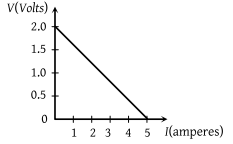(1) 2V, 0.5 Ω

(2) 2 V, 0.4 Ω

(3) > 2 V, 0.5 Ω

(4) > 2 V, 0.4 Ω

Concept Questions :-

Emf and terminal voltage
High Yielding Test Series + Question Bank - NEET 2020

Difficulty Level:

When a current I is passed through a wire of constant resistance, it produces a potential difference V across its ends. The graph drawn between log I and log V will be

(1)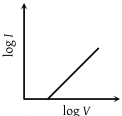(2)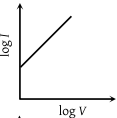(3)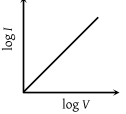(4)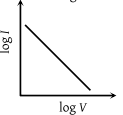Concept Questions :-

Derivation of Ohm's law
High Yielding Test Series + Question Bank - NEET 2020

Difficulty Level:

The V-I graph for a conductor at temperature T1 and T2 are as shown in the figure. $\left({T}_{2}-{T}_{1}\right)$ is proportional to :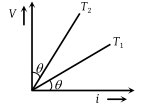(1) $\mathrm{cos}2\theta$

(2) $\mathrm{sin}\theta$

(3) $\mathrm{cot}2\theta$

(4) $\mathrm{tan}\theta$

Concept Questions :-

Current and current density
High Yielding Test Series + Question Bank - NEET 2020

Difficulty Level:

A cylindrical conductor has a uniform cross-section. The resistivity of its material increases linearly from the left end to the right end. If a constant current is flowing through it and at a section distance x from the left end, the magnitude of electric field intensity is E, which of the following graphs is correct :

(1)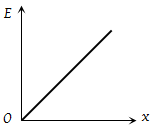(2)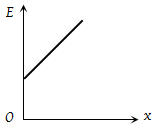(3)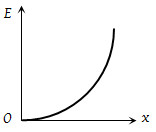(4)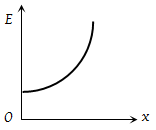Concept Questions :-

Derivation of Ohm's law
High Yielding Test Series + Question Bank - NEET 2020

Difficulty Level:

The V-I graph for a conductor makes an angle θ with V-axis. Here V denotes the voltage and I denotes current. The resistance of the conductor is given by :

(1) $\mathrm{sin}\theta$

(2) $\mathrm{cos}\theta$

(3) $\mathrm{tan}\theta$

(4) $\mathrm{cot}\theta$

Concept Questions :-

Derivation of Ohm's law